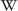# Binary Number

(Redirected from binary)

The binary number is a value system and a special code for computers, which contains only the two numbers 0 (power off) and 1 (power on). A binary number also values of the basis 2.

The construction of the decimal number 2 needs two positions, because 0 and 1 are for the decimal numbers 0 and 1, also must be the decimal number 2 in binary numbers "01". Every new position is another power of 2 (2, 4, 8, 16, 32, ...). With n binary numbers can be constructed 2 to the power of n eventualities.

This table views the construction of the first 16 values of the decimal system (0 until 16) with the first 4 positions with the binary numbers.

Binäre Zahl Dezimalzahl
0 0
1 1
10 2
11 3
100 4
101 5
110 6
111 7
1000 8
1001 9
1010 10
1011 11
1100 12
1101 13
1110 14
1111 15
now 5 positions
10000 16
10001 17
etc. etc.

## Exchanges

Very important is for the programmer the exchange form binary numbers in decimal numbers or the reverse.

• Binary numbers into decimal numbers:

For example this binary number 1011 That must be insert in this formula:

`PRINT "DECIMAL NUMBER: " 1*2↑3 + 0*2↑2 + 1*2↑1 + 1*2↑0`

Screenoutput: DECIMAL NUMBER: 11

• Decimal numbers into binary numbers: 11

The converting of a decimal number into a binary number is a little bit difficult. The easiest way is the method of devision. A decimal number will be devided so long through 2 until the rest is 0. At each step will be noted the rest. For each rest is noted a 1, too. When the 1 is be added backwards (down to up), you get the correct binary number.

```11 : 2 = 5 rest   ...1
05 : 2 = 2 rest   ..1
02 : 2 = 1 rest   .0
01 : 2 = 0 rest   1
----------------------
Binary Number: 1011
```

In computer science didn't exist only the binary system and decimal system, also hexadecimal and many more like a nibble1011 (4 bits).Wikipedia: Binary_numeral_system